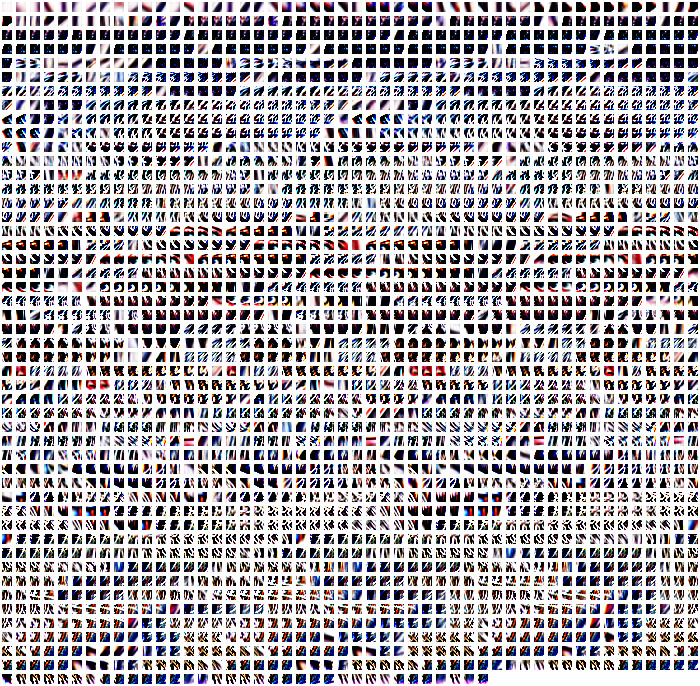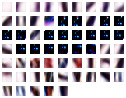# phi transform of lenna

So, it has taken some thinking, coding and computing time, but I now have a phi-transform of the edge-enhanced Lenna. I still don't know if this alley will help with image recognition, and I don't have the computing resources to do large scale image recognition, but I might as well document my work. Considering others have done similar things in the past is a hint that I might be heading in the right direction. eg, my average-categorize has some similarity with sparse coding and vector quantization.

Let's see if I can explain my average categorize in these terms:
```-- start with a set of training superpositions, x_i
-- we desire to find an average-categorized set of superpositions phi_i, also called the dictionary
-- initially we have no dictionary superpositions, so set phi_0 to x_0
-- for each x_i, i > 0:
--- find the k that maximizes simm(x_i,phi_k)
--- if simm(x_i,phi_k) >= t, then set phi_k = phi_k + x_i*simm(x_i,phi_k)
--- else: if simm(x_i,phi_k) < t, then add x_i in to the dictionary
```
Now, let's step through our process and results:
```-- start with this image (small Lenna, edge-enhanced 40):-- map this to a big collection of superpositions, using 10*10 image tiles:
\$ ./create-image-sw.py 10 small-lenna--edge-enhanced-40.png
\$ cd sw-examples
\$ mv image-ngram-superpositions-10.sw small-lenna-edge-40--image-ngram-superpositions-10.sw
\$ cd ..

-- average-categorize with t = 0.7:
\$ ./the_semantic_db_console.py
sa: average-categorize[layer-0,0.7,phi,layer-1]
sa: save small-lenna-edge-40--save-average-categorize--0_7.sw

-- average-categorize with t = 0.4:
\$ ./the_semantic_db_console.py
sa: average-categorize[layer-0,0.4,phi,layer-1]
sa: save small-lenna-edge-40--save-average-categorize--0_4.sw

-- filter these largish sw files into just the layer-1 results:
\$ cd sw-examples
\$ grep "^layer-1 " small-lenna-edge-40--save-average-categorize--0_7.sw > small-lenna-edge-40--layer-1--0_7.sw
\$ grep "^layer-1 " small-lenna-edge-40--save-average-categorize--0_4.sw > small-lenna-edge-40--layer-1--0_4.sw
\$ cd ..

-- visualize the average categories (sw file hard-coded into create-sw-images.py for now):
\$ ./create-sw-images.py
\$ cd work-on-handwritten-digits/
\$ ls -1tr average-categorize-images/* > image-list.txt
\$ montage -geometry +2+2 @image-list.txt small-lenna-edge-40--average-categorize-0_7.jpg

\$ ./create-sw-images.py
\$ cd work-on-handwritten-digits/
\$ ls -1tr average-categorize-images/* > image-list.txt
\$ montage -geometry +2+2 @image-list.txt small-lenna-edge-40--average-categorize-0_4.jpg

-- show the results of that:
k = 10 (ie 10*10 tiles)
t = 0.7
time: 1 week, 2 days
how-many layer-0: 44,100
how-many layer-1: 2,435k = 10
t = 0.4
time: 8 hours, 20 minutes
how-many layer-0: 44,100
how-many layer-1: 59```
So clearly t = 0.7 is too large, and gives way too many categories. Not to mention, very slow! Whether t = 0.4 is the right size, I'm not sure. But it's probably close. And our k is a free parameter too. I'm now thinking k = 10 is too large. Maybe something in the 4 to 7 range?

Now we have the categories, we can do our phi transform. I was going to do it with our t = 0.7 tiles, but I estimate that would take over a month! But here is the result for t = 0.4 (./phi-transform.py 10 small-lenna--edge-enhanced-40.png) at a run time of 12 hours:
For comparison here is the original small Lenna:
And I guess that is the main content I wanted for this post. As for whether this is useful I don't yet know. For comparison I would like to re-run this code but starting with the standard Lenna, instead of the edge-enhanced Lenna. It would be interesting to see if it extracted edges by itself. I suspect it would. For now though, it's probably time I went back to the MNIST digits, and see what I can do with them. I have a couple of new ideas for that, so we will see.

Also, I should mention, I'm certain we won't get decent image recognition with just one layer. The hint for this is the brain, and the deep learning guys. So my task is then to work out how to apply multiple layers of average-categorize, and a multi-layer phi-transform. My hunch is we need to consider larger and larger tile sizes. Above we used 10*10 for the first layer. So maybe map 20*20 tiles to phi superpositions, run average-categorize on that. Then consider say 30*30 tiles, and run that through three layers of average-categorize. That's all a hunch for now. I'll probably apply it to the MNIST data first, and test it a little.

Update: this is what you get after you apply edge-enhance to the above phi-transformed Lenna:

I personally think this image is kind of amazing! That we can go from the original Lenna to this, where the image boundaries scream at you, is quite cool. Though admittedly it took quite a bit of processing and thinking time. Also turns out applying edge enhance to phi-transformed digits is also a really useful normalization step. In early testing it earned me 8% improvement on MNIST just by itself. I'll post on that at some stage.

Home
previous: new tool phi transform
next: averaging out noise

updated: 19/12/2016
by Garry Morrison
email: garry -at- semantic-db.org